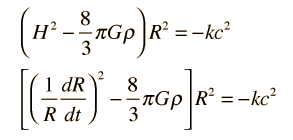The Friedmann Equation

Alexander Friedmann of Russia is credited with developing a dynamic equation for the expanding universe in the 1920s. This was a time when Einstein, Willem de Sitter of the Netherlands, and Georges Lemaitre of Belgium were also working on equations to model the universe. Friedmann developed it as a relativistic equation in the framework of general relativity, but the description here will be limited to a simplified, non-relativistic version based on Newton's laws.

Convenient forms of Friedmann's equation with which to examine the expansion time and temperature for a big bang model of the universe areBesides the density and gravitation constant G, the equation contains the Hubble parameter H, a scaling parameter R, and a factor k which is called the curvature parameter. The curvature parameter indicates whether the universe is open or closed. The above equations do not specify the nature of the density ρ. They do not include any particle interactions other than gravitational attraction. Such particle interactions, like collisions, could be specified in terms of pressure, so the model above is sometimes referred to as a "pressureless" universe. More detailed versions of the Friedman equation include such effects.

Einstein considered adding another term, the famous (or infamous) cosmological constant which would produce a static universe.

 Relate to temperature and expansion time
Index

References
Rohlf
Ch. 19
Kaufmann
Ch. 28

 HyperPhysics***** Astrophysics R Nave
Go Back

The Curvature Parameter

The Friedmann equation which models the expanding universe has a parameter k called the curvature parameter which is indicative of the rate of expansion and whether or not that expansion rate is increasing or decreasing. It indicates the future fate of the universe.

• If k = 0 then the density is equal to a critical value at which the universe will expand forever at a decreasing rate. This is often referred to as the Einstein-de Sitter universe in recognition of their work in modeling it. This k = 0 condition can be used to express the critical density in terms of the present value of the Hubble parameter.

• For k > 0 the density is high enough that the gravitational attraction will eventually stop the expansion and it will collapse backward to a "big crunch". This kind of universe is described as being a closed universe, or a gravitationally bound universe.
• For k < 0 the universe expands forever, there not being sufficient density for gravitational attraction to stop the expansion.

 Relate to temperature and expansion time
Index

References
Rohlf
Ch. 19
Kaufmann
Ch. 28

 HyperPhysics***** Astrophysics R Nave
Go Back

The Cosmological Constant

Einstein proposed a modification of the Friedmann equation which models the expanding universe. He added a term which he called the cosmological constant, which puts the Friedmann equation in the formThe original motivation for the cosmological constant was to make possible a static universe which was isotropic and homogeneous. When the expansion of the universe was established without doubt, Einstein reportedly viewed the cosmological constant as the "worst mistake I ever made". But the idea of a cosmological constant is still under active discussion. Rohlf suggests that the physical interpretation of the cosmological constant was that vacuum fluctuations affected space time. A non-zero value for the cosmological constant could be implied from measurements of the volume densities of distant galaxies, but such measurements give a negative result, showing an upper bound ofThis implies that on the scale of the whole universe, vacuum fluctuation effects cancel out. This assessment comes at a time when theoretical calculations suggest vacuum fluctuation contributions from quarks on the order of 10-6 m-2.

Index

References
Rohlf
Ch. 19
Kaufmann
Ch. 28

 HyperPhysics***** Astrophysics R Nave
Go Back

Critical Density for the Expanding Universe

If the curvature parameter in the Friedmann equation which models the expanding universe has the value k = 0, then the universe will expand forever with a decreasing rate of expansion. Under this condition, the Friedmann equation can be used to express the critical density of matter in the universe in terms of the current value of the Hubble parameter. The present value H0 is commonly called the Hubble constant.It is common practice to express the density in terms of a density parameter Ω which is the ratio of density ρ to the critical density ρc. Currently quoted values are based on the WMAP results. More recent studies were made by the Planck satellite.

 Density of luminous matter Dark matter?
Index

References
Rohlf
Ch. 19
Kaufmann
Ch. 28

 HyperPhysics***** Astrophysics R Nave
Go Back